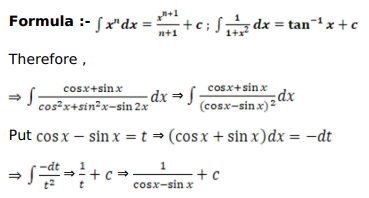# Mark against the correct answer in each of the following:

Question:

Mark $(\sqrt{)}$ against the correct answer in each of the following:

$\int \frac{(\cos +\sin x)}{(1-\sin 2 x)} d x=?$

A. $\log |\sin x-\cos x|+C$

B. $\frac{1}{(\cos x-\sin x)}+C$

C. $\log |\cos x+\sin x|+C$

D. none of these

Solution: